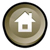Andrei Snarskii, Dmytro Lande, Dmytro Manko
A new method (K-method) of calculating the mutual influence of nodes indirected weight complex networks
// Physica A: Statistical Mechanics and its Applications, 2019. - Vol. 526. - Art. 120899. https://doi.org/10.1016/j.physa.2019.04.135

 Highlights It is possible to use the simplified calculation mechanism, similar to one, is applicable in electrical engineering (the K-method). The proposed algorithm is devoid of the main problems and contradictions inherent in the widely used impulse method. Two new weight characteristics of the concept nodes are proposed, namely pressure and influence as results of accounting for the network structure and .collective interaction.. The results obtained with the help of the K-method correlates well enough with the results obtained by the impulse method. Abstract New method (the K-method) for calculation of characteristics of complex networks is proposed. The method is based on transformation of the initial network and subsequent application of the Kirchhoff rules. The field of application of the method for sparse networks (in which nodes have a cause.effect character of the so-called .cognitive maps.) is proposed. Two new characteristics of concept nodes (.pressure. and .influence.) having a semantic interpretation are proposed. The advantages of the proposed K-method include its computational simplicity (in comparison with other known algorithms) comparable with the task of enumerating subgraphs for sparse networks of relatively small size (in practice - several hundred nodes). At the same time, the results obtained with the help of the K-method for a real network are correlating well enough with the results obtained using the impulse method. Keywords Complex networks; K-method; Mutual influence; Nodes ranking